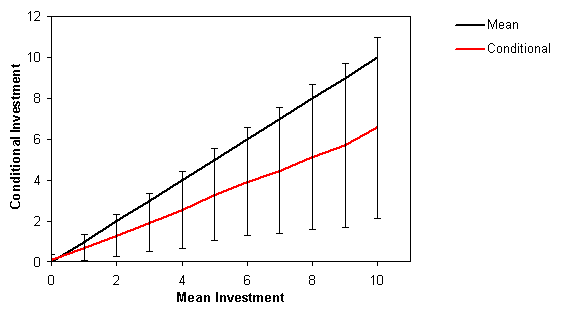## Conditional investment schedules

Instructions

You belong to a randomly formed group of five players. You are given 20 points each and must decide simultaneously on how much to invest to the common pool. The total contribution is multiplied by ½ and added to EACH players payoff - independently of whether this player contributed or not.:
Check:
Question: Suppose that players 1, 2 and 3 give 10 points each and players 4 and 5 contribute nothing. What is the common pool? How many points will each player have?
Answer: 1, 2, and 3 end up with 25 points, the others with 35 points.
Question: What if only player 1 gives 10 points and the others nothing?
Answer: Player 1 ends up with 15 points, the others with 25.
You?ll have to give your conditional investment schedule: How much for each level of average investment by the other three players are you willing to invest?
Evaluations Number of players: 24 (6 games)
Mean unconditional investment: 3.63 ±3.27
Mean conditional investment:Notes
• The readiness to invest increases linearly with the mean investment.
• Conditional investments are significantly lower than the mean investments.

Written by Christoph Hauert